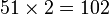# Groups of order 800

## Contents

See pages on algebraic structures of order 800| See pages on groups of a particular order

## Statistics at a glance

The number 800 has prime factors 2 and 5. The prime factorization is:$\! 800 = 2^5 \cdot 5^2 = 32 \cdot 25$

There are only two prime factors of this number. Order has only two prime factors implies solvable (by Burnside's$p^aq^b$-theorem) and hence all groups of this order are solvable groups (specifically, finite solvable groups). Another way of putting this is that the order is a solvability-forcing number. In particular, there is no simple non-abelian group of this order.

Quantity Value Explanation
Number of groups up to isomorphism 1211
Number of abelian groups up to isomorphism 14 (number of abelian groups of order$2^5$)$\times$ (number of abelian groups of order$5^2$) = (number of unordered integer partitions of 5)$\times$ (number of unordered integer partitions of 2) =$7 \times 2 = 14$.
See classification of finite abelian groups and structure theorem for finitely generated abelian groups.
Number of nilpotent groups up to isomorphism 102 (number of groups of order 32)$\times$ (number of groups of order 25 -- see also groups of prime-square order) =$51 \times 2 = 102$.
See number of nilpotent groups equals product of number of groups of order each maximal prime power divisor, which in turn follows from equivalence of definitions of finite nilpotent group.
Number of supersolvable groups up to isomorphism 1126
Number of solvable groups up to isomorphism 1211 There are only two prime factors of this number. Order has only two prime factors implies solvable (by Burnside's$p^aq^b$-theorem) and hence all groups of this order are solvable groups (specifically, finite solvable groups). Another way of putting this is that the order is a solvability-forcing number. In particular, there is no simple non-abelian group of this order.

## GAP implementation

The order 800 is part of GAP's SmallGroup library. Hence, any group of order 800 can be constructed using the SmallGroup function by specifying its group ID. Also, IdGroup is available, so the group ID of any group of this order can be queried.

Further, the collection of all groups of order 800 can be accessed as a list using GAP's AllSmallGroups function.

Here is GAP's summary information about how it stores groups of this order, accessed using GAP's SmallGroupsInformation function:

gap> SmallGroupsInformation(800);

There are 1211 groups of order 800.
They are sorted by their Frattini factors.
1 has Frattini factor [ 10, 1 ].
2 has Frattini factor [ 10, 2 ].
3 has Frattini factor [ 20, 3 ].
4 - 44 have Frattini factor [ 20, 4 ].
45 - 63 have Frattini factor [ 20, 5 ].
64 - 88 have Frattini factor [ 40, 12 ].
89 - 174 have Frattini factor [ 40, 13 ].
175 - 198 have Frattini factor [ 40, 14 ].
199 has Frattini factor [ 50, 3 ].
200 has Frattini factor [ 50, 4 ].
201 has Frattini factor [ 50, 5 ].
202 has Frattini factor [ 80, 49 ].
203 - 215 have Frattini factor [ 80, 50 ].
216 - 230 have Frattini factor [ 80, 51 ].
231 - 236 have Frattini factor [ 80, 52 ].
237 has Frattini factor [ 100, 9 ].
238 has Frattini factor [ 100, 10 ].
239 has Frattini factor [ 100, 11 ].
240 has Frattini factor [ 100, 12 ].
241 - 281 have Frattini factor [ 100, 13 ].
282 - 322 have Frattini factor [ 100, 14 ].
323 - 363 have Frattini factor [ 100, 15 ].
364 - 382 have Frattini factor [ 100, 16 ].
383 has Frattini factor [ 160, 234 ].
384 has Frattini factor [ 160, 235 ].
385 has Frattini factor [ 160, 236 ].
386 has Frattini factor [ 160, 237 ].
387 has Frattini factor [ 160, 238 ].
388 has Frattini factor [ 200, 40 ].
389 - 419 have Frattini factor [ 200, 41 ].
420 - 442 have Frattini factor [ 200, 42 ].
443 - 460 have Frattini factor [ 200, 43 ].
461 - 465 have Frattini factor [ 200, 44 ].
466 - 490 have Frattini factor [ 200, 45 ].
491 - 515 have Frattini factor [ 200, 46 ].
516 - 540 have Frattini factor [ 200, 47 ].
541 - 565 have Frattini factor [ 200, 48 ].
566 - 760 have Frattini factor [ 200, 49 ].
761 - 846 have Frattini factor [ 200, 50 ].
847 - 932 have Frattini factor [ 200, 51 ].
933 - 956 have Frattini factor [ 200, 52 ].
957 - 961 have Frattini factor [ 400, 205 ].
962 - 964 have Frattini factor [ 400, 206 ].
965 - 973 have Frattini factor [ 400, 207 ].
974 - 980 have Frattini factor [ 400, 208 ].
981 - 1011 have Frattini factor [ 400, 209 ].
1012 - 1036 have Frattini factor [ 400, 210 ].
1037 - 1059 have Frattini factor [ 400, 211 ].
1060 - 1064 have Frattini factor [ 400, 212 ].
1065 has Frattini factor [ 400, 213 ].
1066 - 1078 have Frattini factor [ 400, 214 ].
1079 - 1091 have Frattini factor [ 400, 215 ].
1092 - 1104 have Frattini factor [ 400, 216 ].
1105 - 1117 have Frattini factor [ 400, 217 ].
1118 - 1154 have Frattini factor [ 400, 218 ].
1155 - 1169 have Frattini factor [ 400, 219 ].
1170 - 1184 have Frattini factor [ 400, 220 ].
1185 - 1190 have Frattini factor [ 400, 221 ].
1191 - 1211 have trivial Frattini subgroup.

For the selection functions the values of the following attributes
are precomputed and stored:
IsAbelian, IsNilpotentGroup, IsSupersolvableGroup, IsSolvableGroup,
LGLength, FrattinifactorSize and FrattinifactorId.

This size belongs to layer 2 of the SmallGroups library.
IdSmallGroup is available for this size.